# Characterising CSC 2.0

## Introduction

The second major release of the Chandra Source Catalog, CSC 2.0, offers significant improvements over the previous catalog release, CSC 1.1, both in the amount of data included and the analysis procedures followed. CSC 2.0 includes approximately 317,000 unique X-ray sources, roughly three times the number in CSC 1.1, and covers ∼550 deg2 of the sky. The sensitivity limit for compact sources has been significantly improved to ∼5 net counts on-axis for exposures shorter than ∼15 ks. Both the additional data and the improved analysis techniques mandate a full re-characterization of the statistical properties of the catalog, namely, completeness, sensitivity, false source rate, and accuracy of source properties, and we present a summary of that work here. As in CSC 1.1, we use both analysis of real CSC 2.0 catalog results and extensive simulations of blank-sky and point source populations.

## Overall Properties

### Organization of Observations

Source properties are reported at the observation, stack, and master level. CSC 2.0 contains 315,868 compact Master Source records and 1299 extended Master Source records, derived from data in 9,576 separate ACIS and 809 separate HRC observations available in the Chandra Public Archive as of December 31, 2014. Observations with aimpoints within 1′ are co-added into Stacks. All source detection is performed at the stack level. Stacking is done separately for ACIS and HRC observations. There are 7,289 such stacks in CSC 2.0, 6,975 ACIS and 314 HRC . Exposures range from ∼0.6 kiloseconds (ksec) to ∼5.9 megaseconds (Msec), with a median of ∼12 ksec. The distributions of number of observations and total exposure time per stack are shown in Figure 1.

### Figure 1: Observation Stack Histogram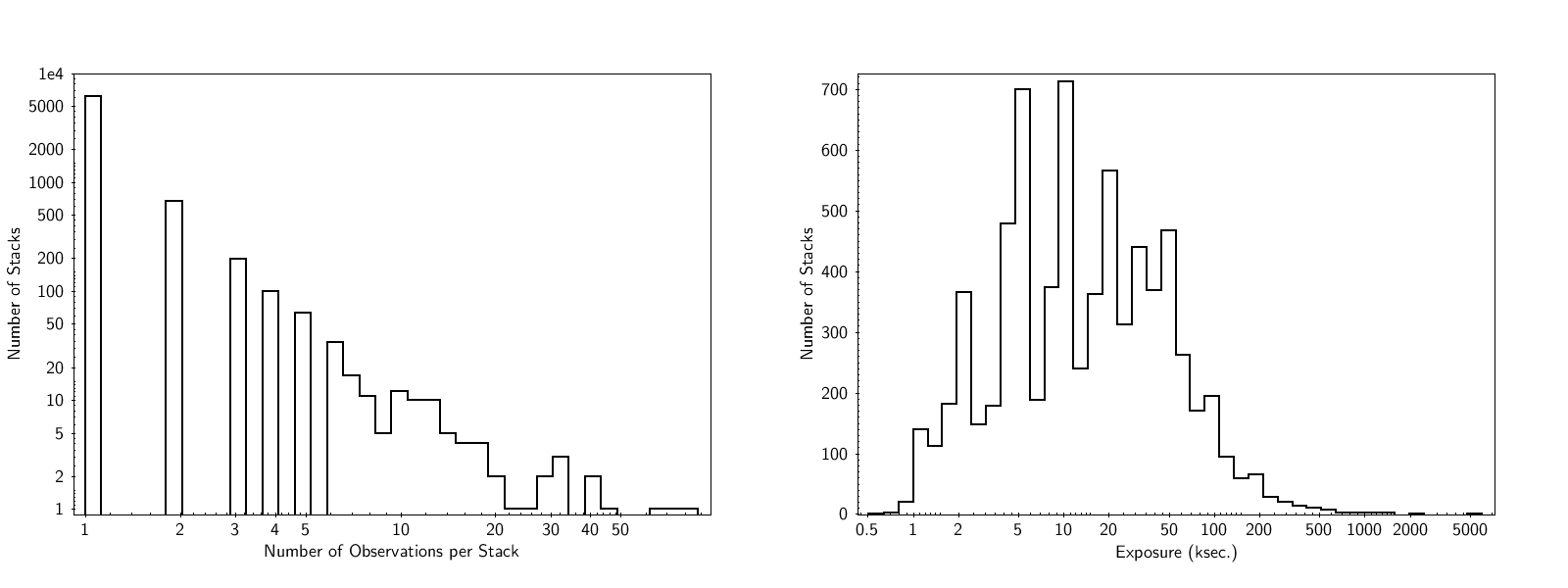### Figure 1: Observation Stack Histogram

Distribution of number of observations per stack (left) and total exposure per stack (right).

At the Master Source level, source properties may include contributions from multiple observations contained in multiple stacks, even if individual observaton aimpoints differ by more than 1′. An example is shown in Figure 2, for master source 2CXO J001120.4-152515. The distribution of the number of stacks contributing to each master source is shown in Figure 3.

### Figure 2: Master Source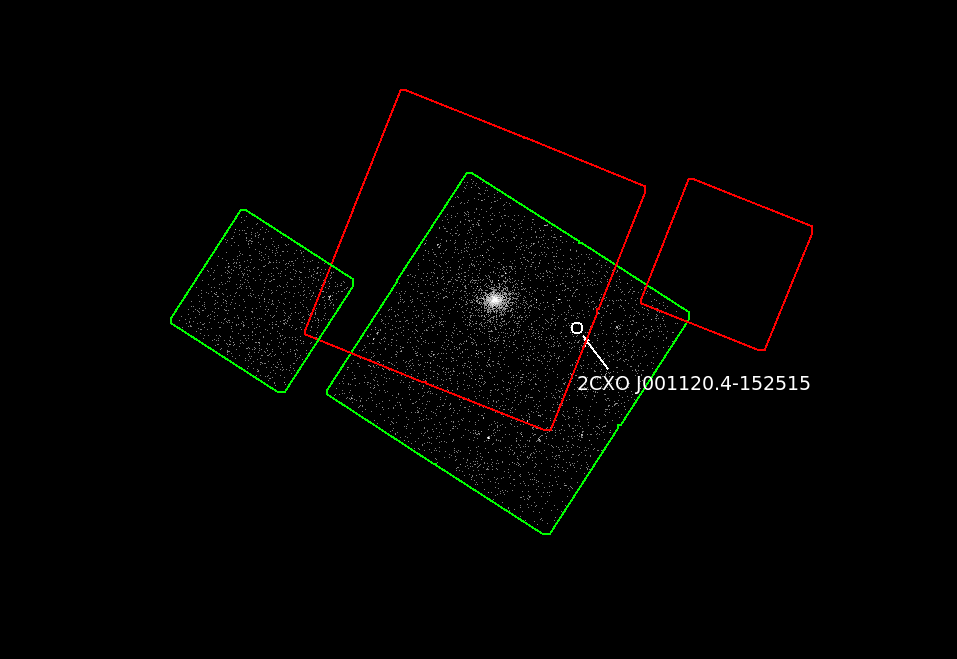### Figure 2: Master Source

Master Source 2CXO J001120.4-152515, indicated by the white circle, includes data both from stack acisfJ0011475m152519_001, with FOV shown in green, and stack acisfJ0011407m152147_001, with FOV shown in red. Events from the first stack only are shown.

### Figure 3: Stacks per Master Source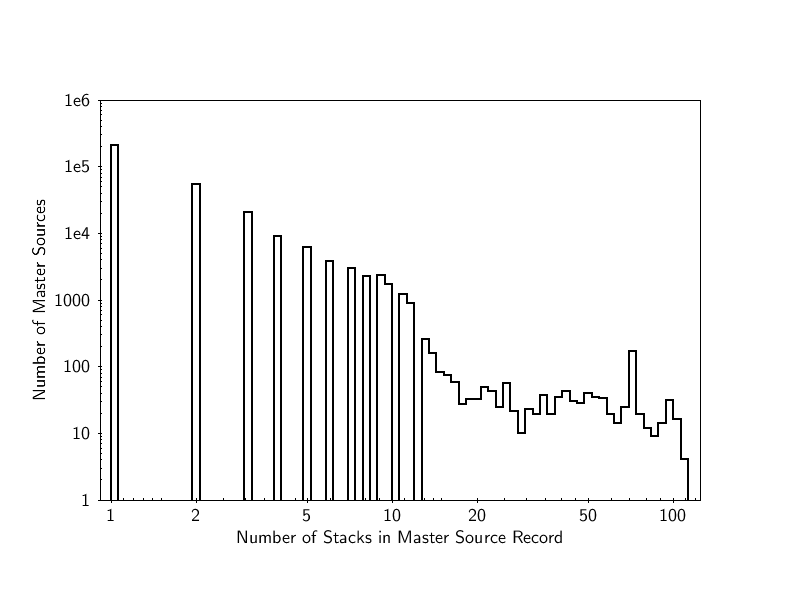### Figure 3: Stacks per Master Source

Distribution of number of stacks contributing to each master source.

Because master sources may be located at different off-axis angles in different stacks, source data quality may vary from stack to stack. In particular, a source detected in one stack at a large off-axis angle may resolve into multiple sources at smaller off-axis angles in another stack. Such "ambiguous" detections will remain linked to master sources in the database, but only data from unambiguous detections will be used to derive master source properties.

Because of the variable source quality in different observations contributing to a master source, and because many X-ray sources are intrinsically variable, we use a Bayesian Blocks algorithm (c.f. "Combining Aperture Photometry Results from Multiple ObsIDs" and Scargle et al. 2013, ApJ 764 167) to group observations into blocks. In each block, a constant flux is consistent with all individual observation level fluxes. ACIS and HRC observations are grouped into separate blocks, and in ACIS blocks, a constant flux must be consistent with all observations in all energy bands. An example is shown in Figure 4. The block with the longest total exposure is selected as the "best" block, and results from it are reported in the Master Source record's aperture photometry quantities.

### Figure 4: Observation MPDFs of Master Source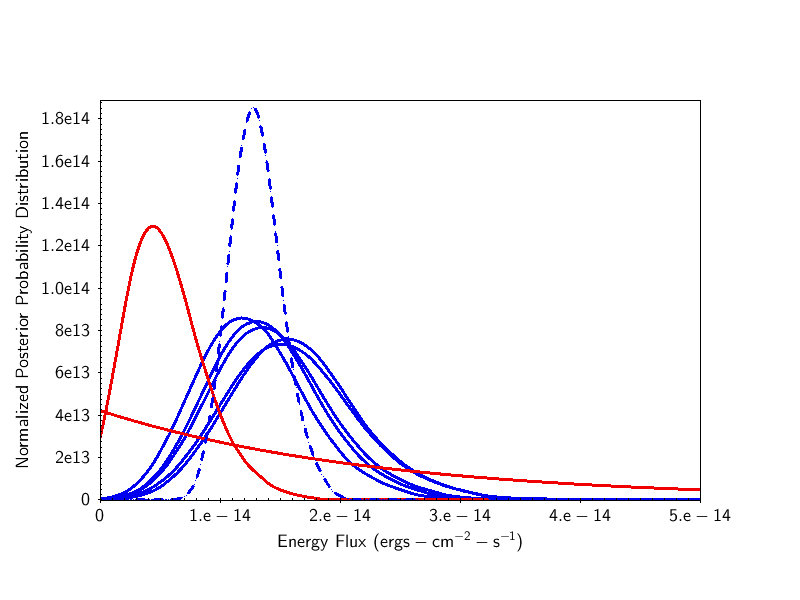### Figure 4: Observation MPDFs of Master Source

Marginalized probability distributions (MPDFs) for w-band energy flux in 7 observations contributing to master source 2CXO J180122.8-254529. Solid curves represent the MPDFs for the source in individual observations. Curves in blue are grouped into the "best" block. The dashed curve is the master source MPDF, which combines data from all observations in the best block.

### Distribution on Sky

The distribution of CSC 2.0 stacks on the sky is shown in Figure 5. As suggested in Figures 1 and 3, in most areas of the sky, stacks include only a few observations. However, several targets, such as the Galactic Center and M31, have been observed repeatedly, resulting in a large number of master sources from many observations and stacks.

### Figure 5: Stacks in the Sky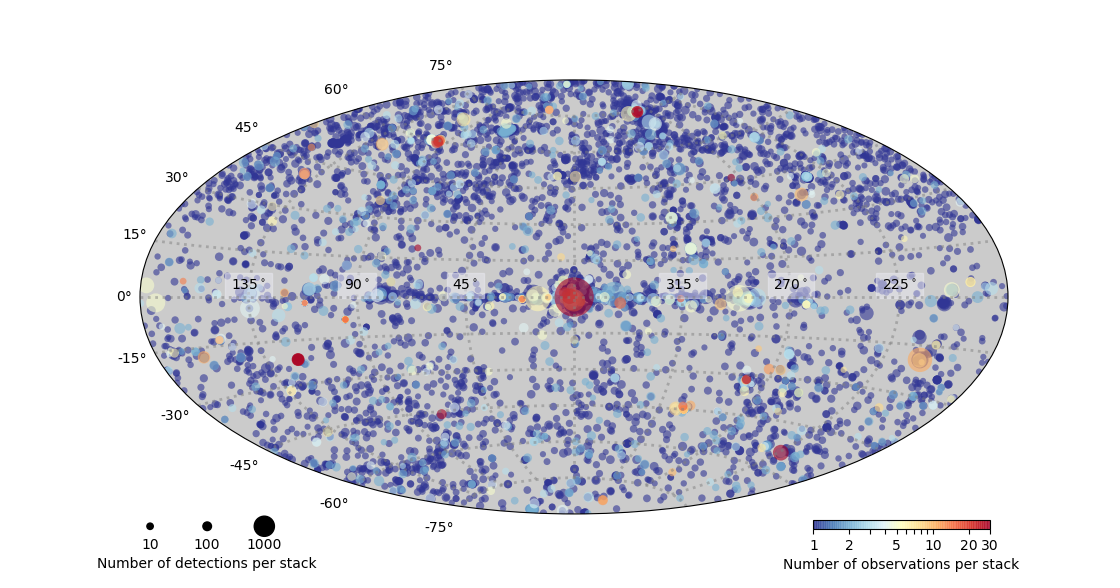### Figure 5: Stacks in the Sky

Distribution of CSC 2.0, stacks on the sky, in galactic coordinates. The dot size indicates the number of sources detected in the stack, and dot color indicates the number of observations.

### Flux Distribution

CSC 2.0 fluxes range from below 10-18 erg cm-2 sec-1 (for the deepest exposures) to 10-10 erg cm-2 sec-1; most sources have fluxes of 10-15–10-13 erg cm-2 sec-1 (b-band, or 0.5-7.0 keV). The distribution of master source fluxes as shown in Figure 6.

### Figure 6: Master Flux Distribution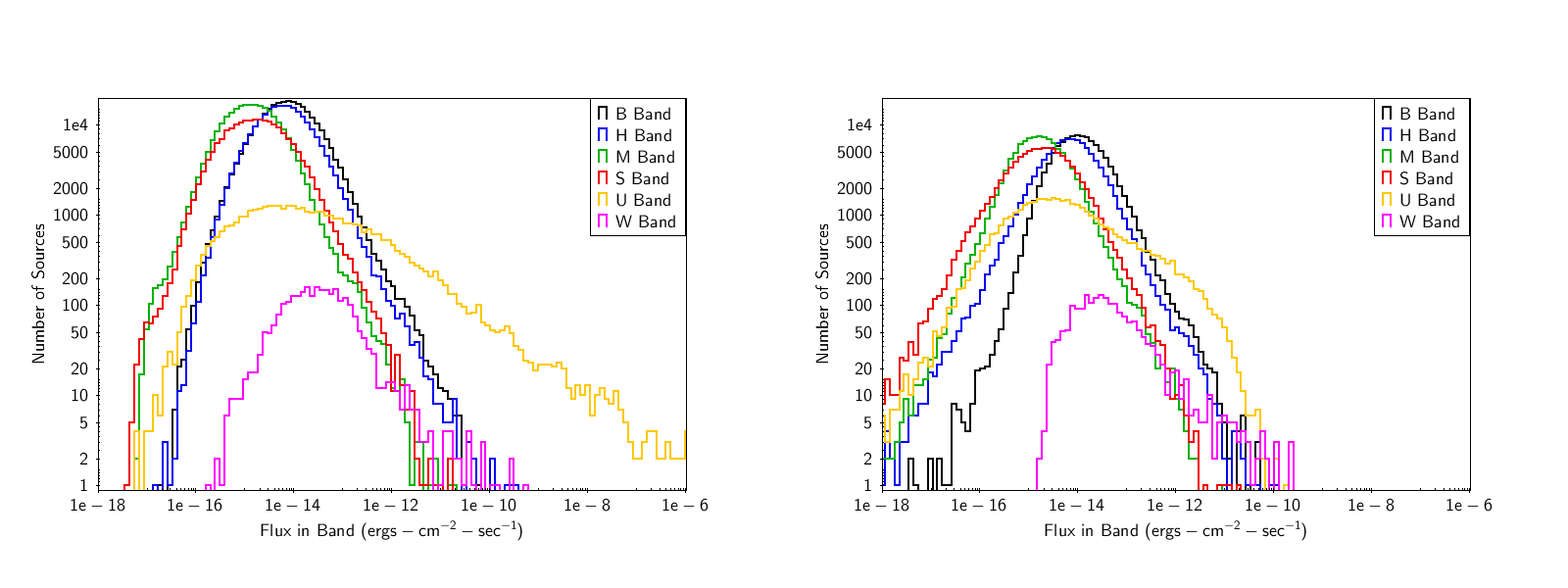### Figure 6: Master Flux Distribution

Distribution of master source fluxes for CSC 2.0 (left) and CSC 1.1 (right).

Although it appears that the CSC 1.1 distributions extend to lower fluxes, it should be noted that the definition of master flux (i.e., flux_aper_〈band〉 quantities) has changed in CSC 2.0. Whereas CSC 1.1 master fluxes were simple averages over fluxes from all contributing observations in a source, in CSC 2.0 they correspond to the flux from the 'best' flux block, i.e., the group of observations with the longest exposure, and in which the individual observation fluxes are consistent with a constant flux across all bands. Moreover, the treatment of upper limits has changed in CSC 2.0, with flux_aper values for upper limits set to 0.0. These sources do not appear in Figure 6 due to the use of log scales.

A more detailed comparison of CSC 1.1 and CSC 2.0 flux distributions is shown in Figure 7 and demonstrates an improved CSC 2.0 sensitivity to fluxes below 10-14 erg cm-2 sec-1.

### Figure 7: Master Flux Distribution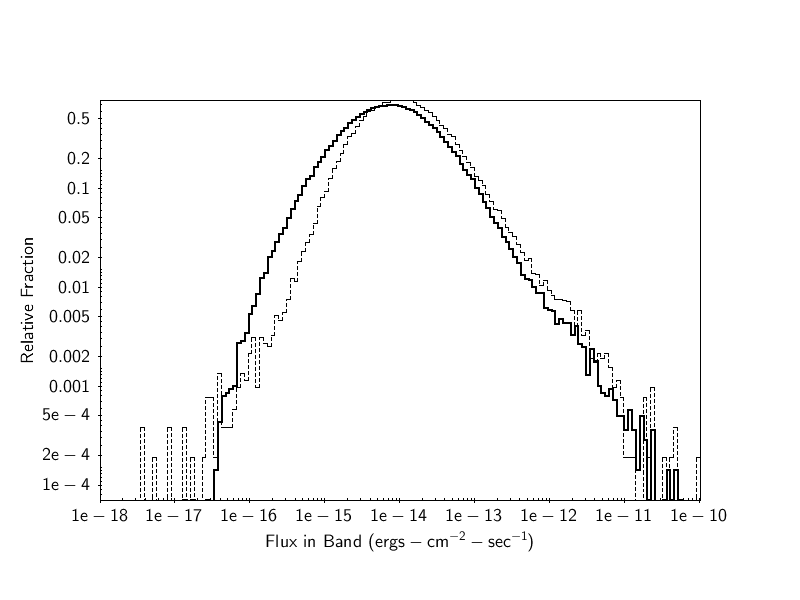### Figure 7: Master Flux Distribution

Histograms of master fluxes for CSC 2.0 (thick, solid line) and CSC 1.1 (thin, dotted line), normalized to unit area.

### Field Background

We compute simple estimates of background, averaged over the field, for each observation in CSC 2.0, by computing the total number of events per detector or chip, and subtracting the total number of source counts provided by aperture photometry. We exclude observations with known extended emission from the analysis. Results are shown in Figure 8 and reveal the expected variation with solar cycle. For ACIS observations, b-band values range from ∼0.2-0.3 counts sec-1 chip-1 for the I3 chip and ∼0.4-0.5 counts sec-1 chip-1 for the S3 chip. For HRC-I observations, values range from ∼25-75 counts sec-1.

### Figure 8: Average Field Background Rates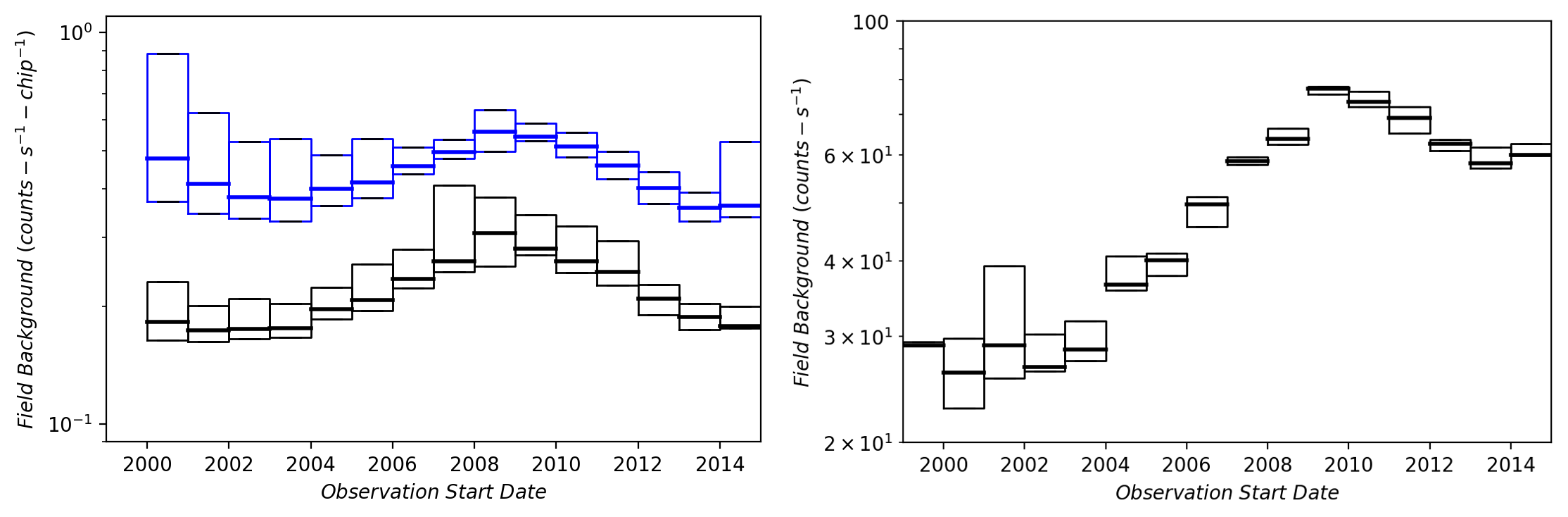### Figure 8: Average Field Background Rates

Average field background rates per detector or chip for ACIS b-band (left) and HRC (right) observations, as a function of observation date. In each year bin, boxes represent the inter-quartile range (25%-75%) of the distribution of background rates, and the thick horizontal lines indicate the medians. For ACIS, the rates per chip for the front-illuminated chip I3 (black) and back-illuminated S3 (blue) are shown. Bins include typically ∼200 observations for ACIS and ∼40 for HRC.

## Limiting Sensitivity and Sky Coverage

### Limiting Sensitivity Maps

The limiting sensitivity maps are computed for each stack in all source detection energy bands. The maps are based on stack-level background maps and represent the minimum point source photon flux, $$p_{min}$$, in units of photons s-1 cm-2 satisfying the inequality:

$P\left(T \ge B + 0.9 p_{min} E | B\right) \lt P^{*}$

where $$P$$ is the cummulative Poisson probability of obtaining more than $$B + 0.9 p_{min} E$$ counts in a 90% ECF aperture with expected background $$B$$ and average exposure $$E$$ in units of cm2 s count photon-1. $$P^{*}$$ is a threshold probability that corresponds to the source detection likelihood threshold, $$\mathcal{L}^{*}=-2\ln{P}$$.

The limiting sensitivity map consists of a single FITS format file for each set of stacked observation detections and science energy band includeing two images, one corresponding to less restrictive likelihood thresholds for sources classified as MARGINAL, and one for a more restrictive threshold for sources classified as TRUE. The MARGINAL and TRUE source detection likelihood thresholds correspond to false source rates of ∼1 and ∼0.1 false sources per stack, respectively, and are determined from simulations. The file is named: 〈i〉〈s〉〈stkpos〉_〈stkver〉N〈v〉_〈b〉_sens3.fits

Here, 〈i〉 is the instrument designation; 〈s〉 is the data source; 〈stkpos〉 is the position component of the stack name, formatted as "Jhhmmsss{p|m}ddmmss"; 〈stkver〉 is the 3-digit version component of the stack name, formatted with leading zeros; 〈v〉 is the data product version number, formatted with leading zeros; and 〈b〉 is the energy band designation.

### Figure 9: ACIS Sensitivity Map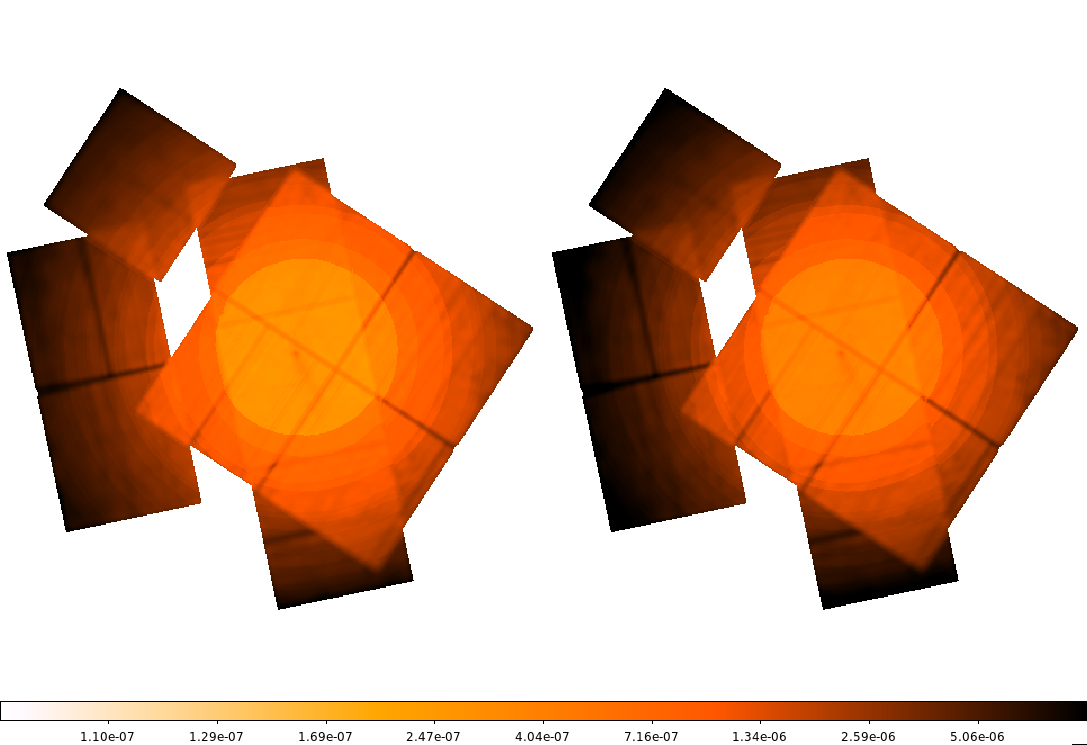### Figure 9: ACIS Sensitivity Map

b-band limiting sensitivity maps for stack acisfJ1509253m585033_001, for MARGINAL (left) and TRUE (right) false source rates.

It should be noted, however, that CSC Release 2.0 source detections are not based on likelihoods derived from Poisson fluctuations, like those in the prior inequality describing the sensitivity maps. Rather, the detection procedure is based on fitting a point source model to image data in the vicinity of candidate sources. For each candidate two 2D spatial models are fit—one consisting of background only, and the other of background plus a point source convolved with the PSF. The best-fit $$C$$-statistic for each model is computed and the probability $$P$$ of obtaining an increase in $$C$$ at least as large as that observed, in the absence of a real source, is evaluated. The source detection likelihood $$\mathcal{L}$$ is computed from this probability.

For the purposes of computing the sensitivity maps, we chose not to use these likelihoods, since that would require constructing PSFs for each sensitivity map pixel (∼4″⨯4″ for ACIS, ∼2″⨯2″ for HRC). Rather we used the simpler aperture quantities described in the inequality, under the assumption that for real point sources, the flux associated with a likelihood derived from aperture quantities is related to the actual flux of a source detected at the source detection likelihood threshold, i.e.,

$p_{min}\left(\mathcal{L}_{fit}\right) \propto F\left(\mathcal{L}_{fit}\right)$

To calibrate this relation, we selected a sample of isolated CSC Release 2.0 point sources and calculated $$p_{min}$$ from the available aperture quantities, using the actual detection likelihoods. We then compared these to actual photon fluxes and energy fluxes, as reported in the corresponding photflux_aper90 or flux_aper90 columns. Results for the b-band are shown in Figure 10.

### Figure 10: Aperture Fluxes vs. Source Detection Likelihoods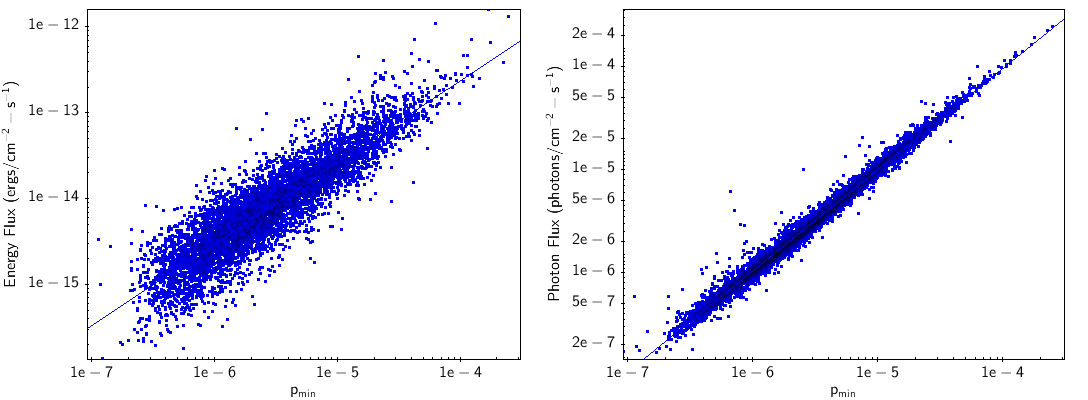### Figure 10: Aperture Fluxes vs. Source Detection Likelihoods

Comparison of flux_aper90_b (left) and photflux_aper90_b (right) values vs. $$p\_{min}$$, determined using the sources' detection likelihoods, for a sample of isolated point sources.

For all bands, we find the data are well-fit with relations of the form:

$\log_{10}{\left(flux\ \mathrm{or}\ photon\ flux\right)} = m\log_{10}{\left(p_{min}\right)} + c \ .$

Values of $$m$$ and $$c$$ are given in the table below, and may be used to correct sensitivity map values to true limiting sensitivities, in either energy flux or photon flux, as the detection likelihood thresholds.

#### Table 1

Band Energy Flux Photon Flux
m c m c
b 0.960 -8.781 0.993 -0.034
s 1.028 -8.595 0.988 -0.049
m 0.983 -8.701 0.988 -0.053
h 0.993 -8.222 0.990 -0.057
w 0.950 -8.896 0.952 -0.264

### Sky Coverage

In addition to stack-level sensitivity maps, all-sky maps of limiting sensitivity are constructed by regridding corrected individual maps in HEALPix nested celestial grid with index=16 $$\left(\theta_{pix} \approx 3.22^{\prime\prime}\right)$$. An example HEALPix map for stack acisfJ1509253m585033_001 is shown in Figure 11.

### Figure 11: HEALPix Map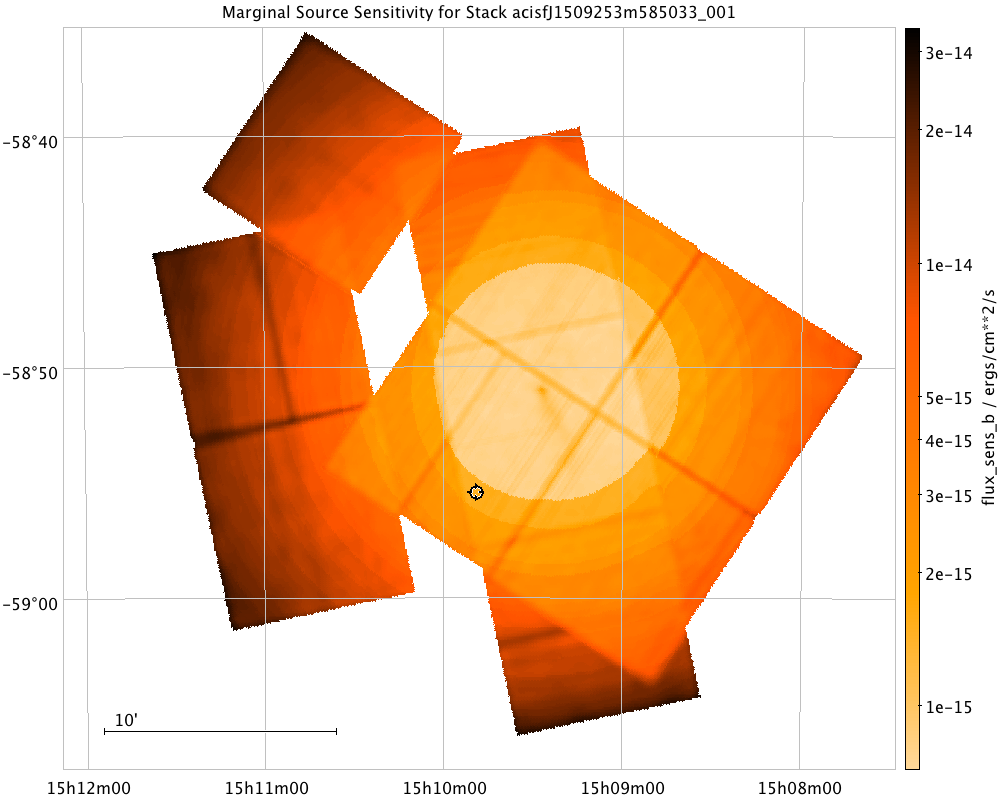### Figure 11: HEALPix Map

b-band HEALPix map for stack acisfJ1509253m585033_001, for MARGINAL false source rates.

All populated HEALPix pixels are collected in the catalog database. If a particular HEALPix pixel occurs in multiple stacks, the highest sensitivity value (i.e., lowest sensitivity value) is used. Users may then query the database for limiting sensitivity values near positions of interest. All-sky maps are generated for all detection energy bands (s, m, h, b, w), for both MARGINAL and TRUE detection thresholds. The total cumulative sky coverage at TRUE detection thresholds is ∼520 deg2 for b-band and ∼55 deg2 for the w-band, and is shown as a function of energy flux in Figure 12.

### Figure 12: Cummulative Sky Coverage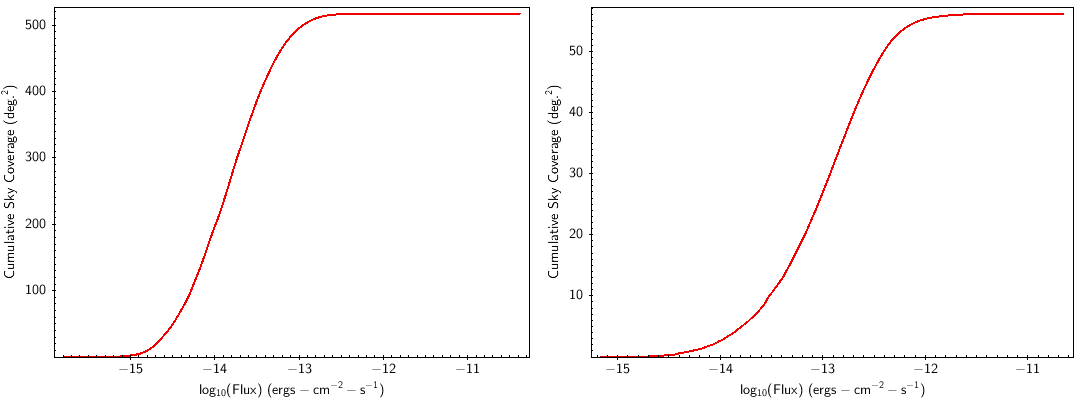### Figure 12: Cummulative Sky Coverage

Cumulative sky coverage for b-band (left) and w-band (right), for TRUE detection thresholds.

## Source Detection

Source detection in CSC 2.0 is a two-step process. After observations have been co-added into stacks, the combined image data are analyzed with two separate source detection tools—the CIAO tool wavdetect and a Voronoi Tesselation based detection tool, mkvtbkg, developed by the CSC team for detecting large extended sources and point sources embedded in diffuse emission. Both tools are run with very low detection thresholds to maximize the number of real sources detected. A point source model is fit to combined image data for all source candidates, and candidates are classified as FALSE, MARGINAL, or TRUE, depending on where their detection likelihoods fall with respect to two likelihood thresholds, corresponding to false source rates of ∼1 (FALSE-MARGINAL boundary) and ∼0.1 (MARGINAL-TRUE boundary) false sources per stack, respectively.

Thresholds are determined using simulations in which the event lists for actual catalog observations are replaced with blank-sky event lists derived from the background map for the corresponding observation, randomized with Poisson noise. Typically, ∼100-200 runs of the same simulation set were generated. A list of simulation sets used is given in Table 2.

#### Table 2

Aimpoint ObsIDs Tstack (ksec) Marginal Source Detections True Source Detections
Detections (runs) FSR Detections (runs) FSR
ACIS-I 15164 9 40 (225) 0.18) 3 (225) 0.01
ACIS-I 14024 135 59 (194) 0.30 1 (194) 0.01
ACIS-I 3251, 10413, 10786, 10797 135 82 (153) 0.54 25 (153) 0.16
ACIS-I 14022, 14023 296 64 (158) 0.41 8 (158) 0.05
ACIS-S 7921 135 100 (199) 0.50 33 (199) 0.17
ACIS-S 11688, 11689, 12106, 12119 288 223 (178) 1.25 33 (178) 0.19
ACIS-S 11688, 11689, 12106, 12119 288 60 (178) [no chip8] 0.34 20 (178) [no chip8] 0.11

These blank-sky observations are then processed in the standard catalog detection pipeline, and the resulting detections analyzed as a function of likelihood, background density, exposure, and detector configuration to derive the FALSE-MARGINAL and MARGINAL-TRUE likelihood threshold functions (see, e.g., "ACIS False Source Likelihood Thresholds").

### False Source Rate

We can demonstrate the performance of the likelihood threshold functions by computing the actual false source rates in the various simulation runs. An example simulated event list from the four-ObsID ACIS-I simulation set is shown in Figure 13, and the distribution of likelihoods vs. off-axis angle is shown in Figure 14. For this simulation set, we find 82 detections with likelihoods above the FALSE-MARGINAL threshold, yielding an average false source rate of 0.54+/-0.06 per field for MARGINAL sources. Similarly, we find 25 detections above the MARGINAL-TRUE threshold, for an average false source rate of 0.16+/-0.03 per field for TRUE sources.

Calculated false source rates for all the simulation sets in Table 2 are given in the table. In general, all are consistent with desired rates of 1 false source per field for MARGINAL sources and 0.1 per field for TRUE sources, with the exception of the ACIS-S aimpoint four-ObsID set. We note that, as in CSC 1.1 there is an excess of detections in the vicinity of bad columns in Chip 8, as shown in Figure 15. If these detections are excluded, the false source rates for this simulation set agree with those in the other sets.

### Figure 13: ACIS-I Four_ObsID Stack Simulation Set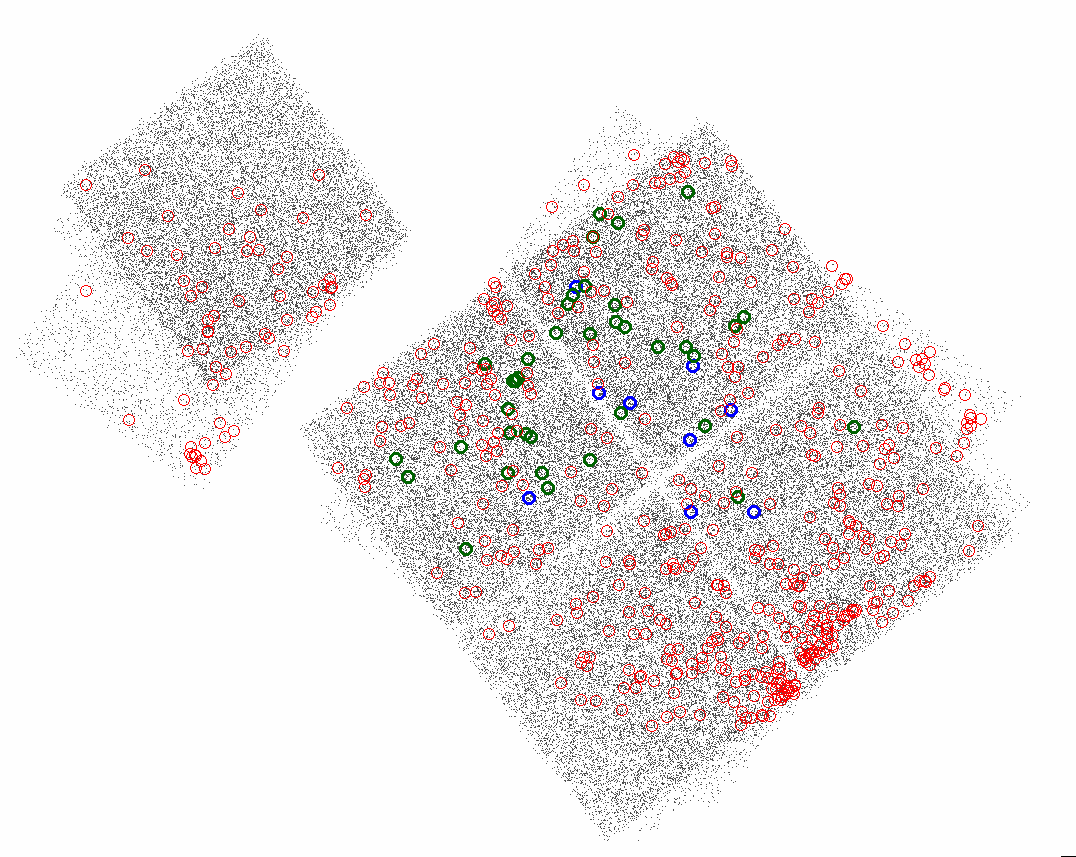### Figure 13: ACIS-I Four_ObsID Stack Simulation Set

Example simulated event list for a 4-ObsID stack with ACIS-I aimpoint and stack exposure of ∼135 kiloseconds. FALSE (red), MARGINAL (green), and TRUE (blue) sources for all runs of this simulation are indicated.

### Figure 14: Detection Likelihoods from ACIS-I Four_ObsID Stack Simulation Sets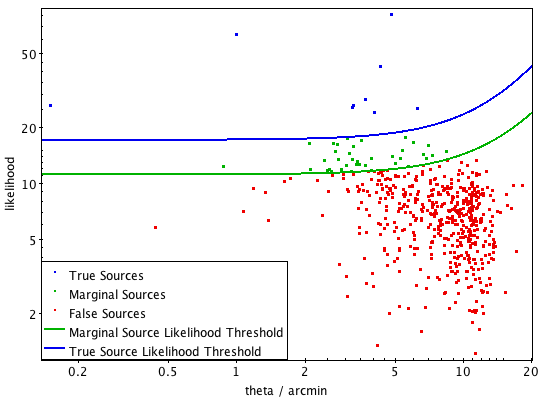### Figure 14: Detection Likelihoods from ACIS-I Four_ObsID Stack Simulation Sets

Detection likelihoods for sources detected in ∼150 simulation reuns of a 4-ObsID stack with ACIS-I aimpoint and stack exposure of ∼135 kiloseconds. FALSE (red), MARGINAL (green), and TRUE (blue) sources for all runs are shown.

### Figure 15: ACIS-S Four-ObsID Stack Simulation Set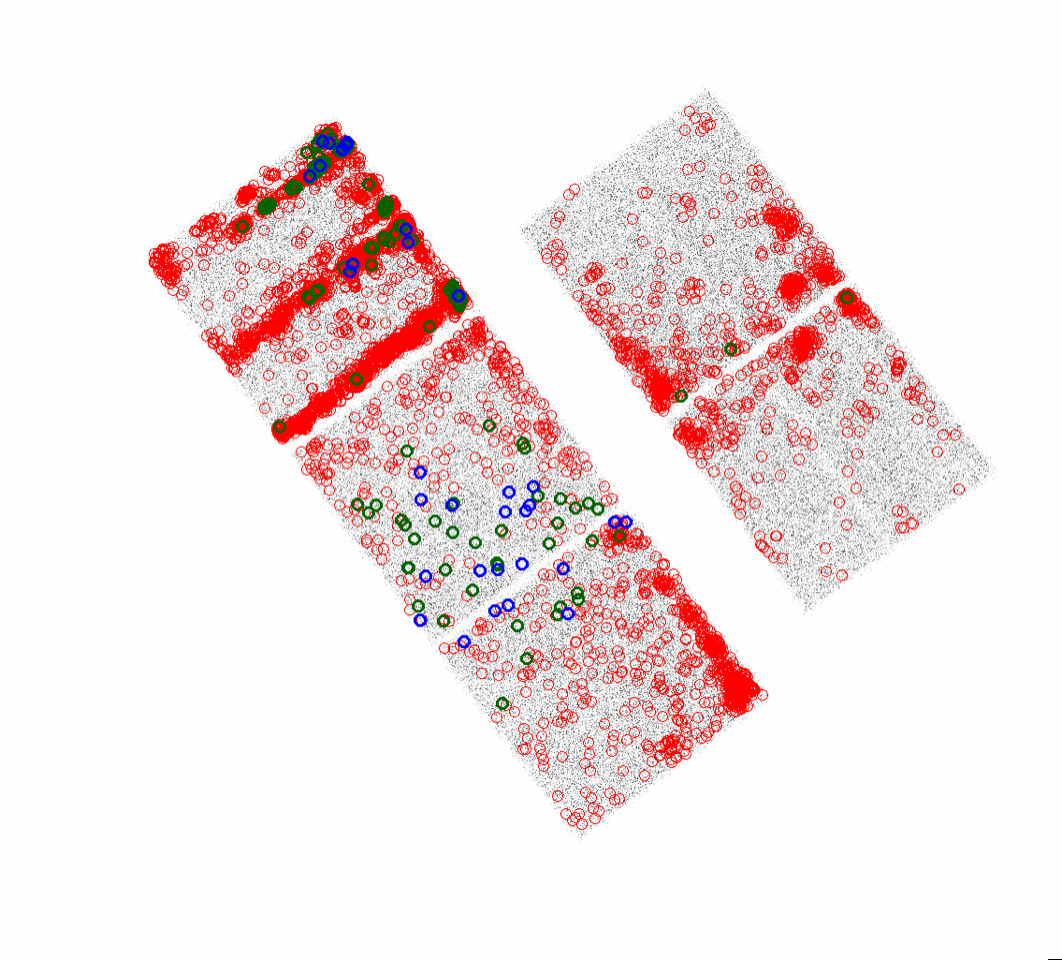### Figure 15: ACIS-S Four-ObsID Stack Simulation Set

The ACIS-S 4-ObsID stack simulation set shows an excess of detections in Chip 8. False (red), MARGINAL (green), and TRUE (blue) sources for all runs of this simulation are indicated.

### Detection Efficiency

We estimate the detection efficiency in CSC 2.0, by comparing the number of source detections in individual observations that are part of the Chandra Deep Field South Survey to the number of sources reported in that survey's 7 Msec catalog (Luo et al., 2017 ApJS 228 2). The CDFS sources are derived from an analysis of stacked Chandra ACIS-I images, totaling ∼7 Msec., and so can be considered complete at the exposures of individual observations. We have selected three individual ACIS-I observations, ObsIDs 12047, 12054 and 17535, with exposures of ∼10, ∼60, and ∼120 ksec, respectively. We extracted the CDFS sources which lie in the fields-of-view of each of these observations and constructed histograms of fluxes in the CDFS 'full' band (0.5-7.0 keV). We then constructed similar histograms using only CDFS sources which would be classified as MARGINAL or TRUE in the CSC 2.0, source detection lists for those observations. The ratio of the two distributions provides estimates of the detection efficiency. Examples of detection efficiency curves for sources in two ranges of off-axis angle, $$0 < \theta \leq 6^{\prime}$$ and $$\theta > 6^{\prime}$$, are shown in Figure 16.

### Figure 16: Detection Efficiency vs. Flux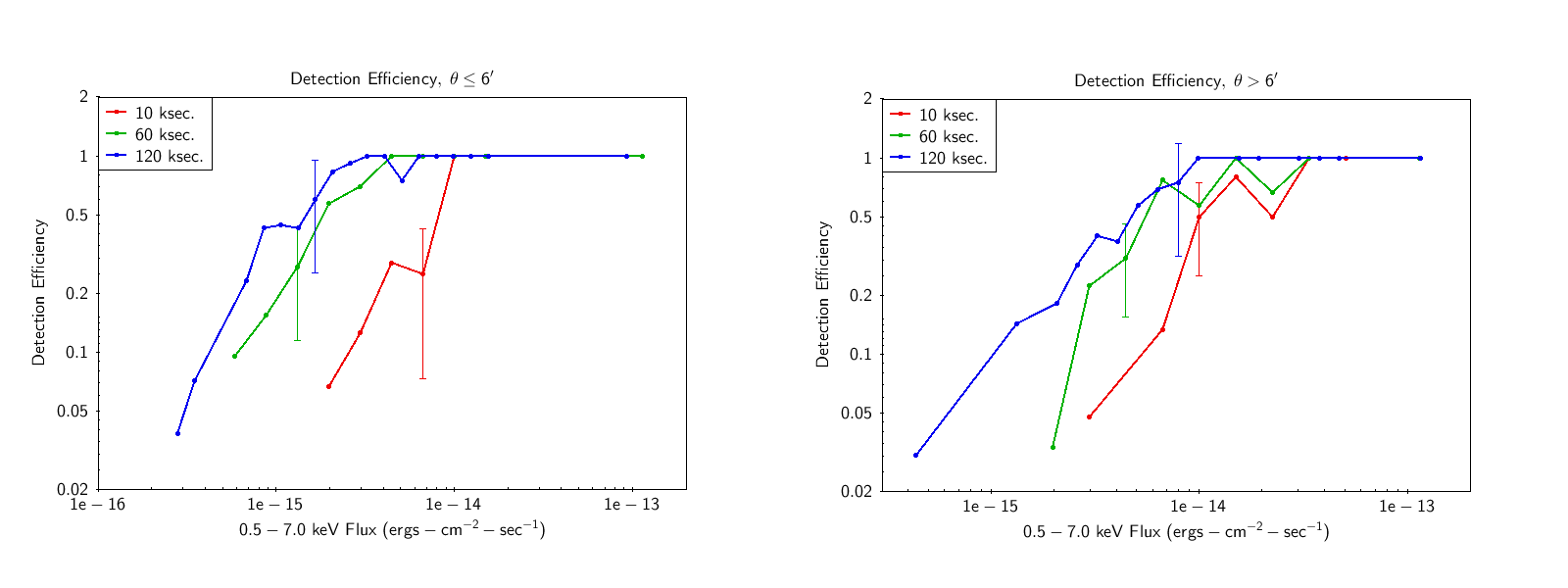### Figure 16: Detection Efficiency vs. Flux

Detection Efficiency for ACIS-I observations of ∼10, ∼60, and ∼120 ksec. Typical error bars are indicated for each curve.

## Astrometry

To characterize the astrometric accuracy of CSC 2.0, we cross-match CSC 2.0, Master Source positions with positions of stars in the SDSS-DR13 catalog, using a technique similar to that used in CSC 1.1, (Rots & Budavári, 2011 ApJS 192 8). A histogram of angular separation $$\delta$$ for a preliminary sample of $$\sim\,12000$$ unambiguous matches is shown in Figure 17. By considering only CSC 2.0, sources which derive from a single observation, we can investigate the dependence of astrometric accuracy on off-axis angle, $$\theta$$. A plot of $$\delta$$ vs. $$\theta$$ for a sub-sample of $$\sim\,9000$$ single-observation matches is shown in Figure 18. The mean offset is $$\sim\,0.32^{{\prime}{\prime}}$$ for sources with $$\theta<3^{\prime}$$, $$\sim\,0.83^{{\prime}{\prime}}$$ for sources with $$\theta<10^{\prime}$$, and $$\sim\,1.2^{{\prime}{\prime}}$$ overall. We note these values are slightly larger than the corresponding values for CSC 1.1.

### Figure 17: Angular Separation Distribution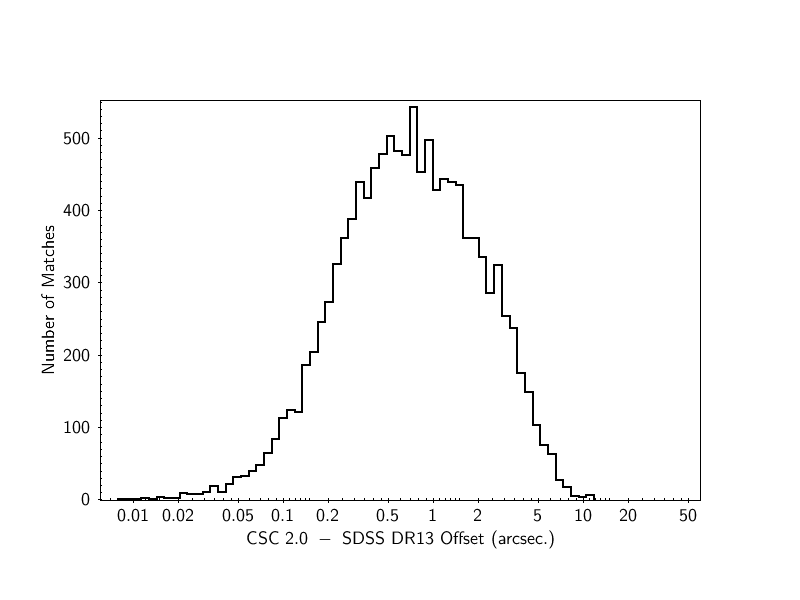### Figure 17: Angular Separation Distribution

Distribution of CSC 2.0-SDSS angular separations for CSC 2.0 sources identified with stars from the SDSS-DR13 Catalog.

### Figure 18: Angular Separation vs. Off-Axis Angle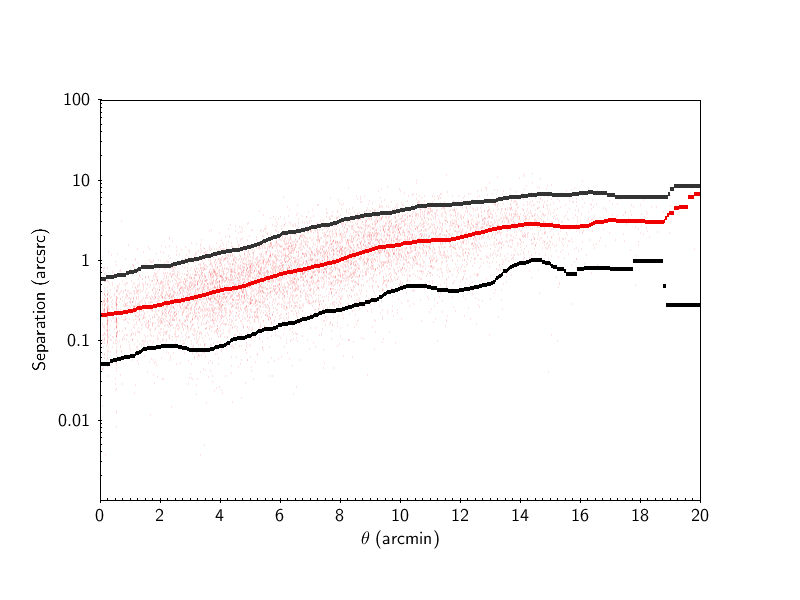### Figure 18: Angular Separation vs. Off-Axis Angle

Angular separation between CSC 2.0 sources and SDSS stars for $$\sim\,9000$$ single-observation matches, as a function of off-axis angle $$\theta$$. Solid black lines indicate 5% and 95% quantiles, and the solid red line indicates the median.

We investigate possible systematic astrometric errors in CSC 2.0, using the same technique used in CSC 1.1, (Rots & Budavári, 2011 ApJS 192 8). We compute normalized separations,

$Z=\delta/\sigma_{tot}\,;\, \sigma_{tot}=\sqrt{\sigma_{CSC}^2+\sigma_{SDSS}^2+\sigma_{sys}^2}$

and examine the distribution of $$Z$$ as different values of systematic error $$\sigma_{sys}$$ are chosen. In principle, $$Z$$ should follow a Rayleigh Distribution. An example is shown in Figure 19. We also bin the sample into multiple bins of $$\sigma_{tot}$$, with comparable number of points $$n$$. For each bin, we compute a reduced $$\chi^2$$

$\chi^{2}=\sum{Z^{2}}/(n-1)$

In principle, the values of $$\chi^{2}$$ should be comparable in all bins.

By varying $$\sigma_{sys}$$ and examining the effect on the distribution of $$Z$$ and $$\chi^{2}$$, we determine that a best value of $$\sigma_{sys}=0.29^{{\prime}{\prime}}$$ for CSC 2.0. We note, again, that this value is larger than the value of $$\sigma_{sys}=0.16^{{\prime}{\prime}}$$ used for CSC 1.1.

### Figure 19: Normalized Angular Separations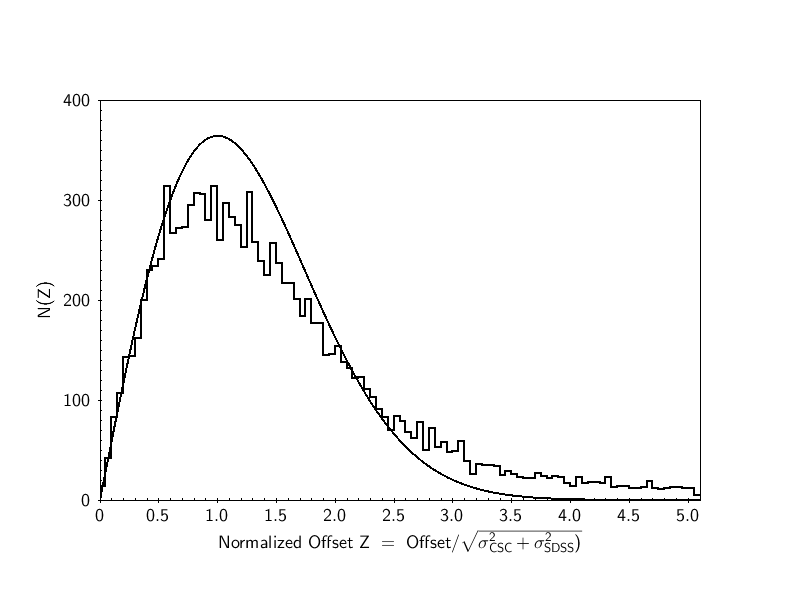### Figure 19: Normalized Angular Separations

Normalized CSC 2.0-SDSS angular separations, assuming no systematic astrometric error. The solid black line is a Rayleigh Distribution, normalized to the same number of points.

## Flux Accuracy

We can provide only a preliminary assessment of the accuracy of fluxes determined from aperture photometry in CSC 2.0, because the generation and analysis of simulated point source data sets with a wide range of input fluxes is not yet complete. Until then, we use CSC 1.1 fluxes to characterize the flux accuracy of CSC 2.0. We limit our analysis to CSC 2.0 sources whose properties are derived exclusively from observations included in CSC 1.1. We find ∼82,000 CSC 2.0 master sources in this sample, and ∼37,000 in a 'high-significance' sub-sample in which the CSC 1.1 flux significance is 5 or greater and the CSC 2.0 likelihood classification is TRUE.

For both samples, we compare the CSC 1.1 master source flux_aper_〈band〉 values with the corresponding CSC 2.0 master source flux_aper_avg_〈band〉 values, since the latter use data from all observations contributing to the master source, as in CSC 1.1, whereas the CSC 2.0 flux_aper_〈band〉 values include only those observations in the best block. A comparison of the b-band fluxes is shown in Figure 20. In general, the CSC 1.1 and CSC 2.0 fluxes are in good agreement, although there appears to be a significant number of sources with lower CSC 2.0 fluxes.

### Figure 20: CSC 2.0 vs. CSC 1.1 Master Source Fluxes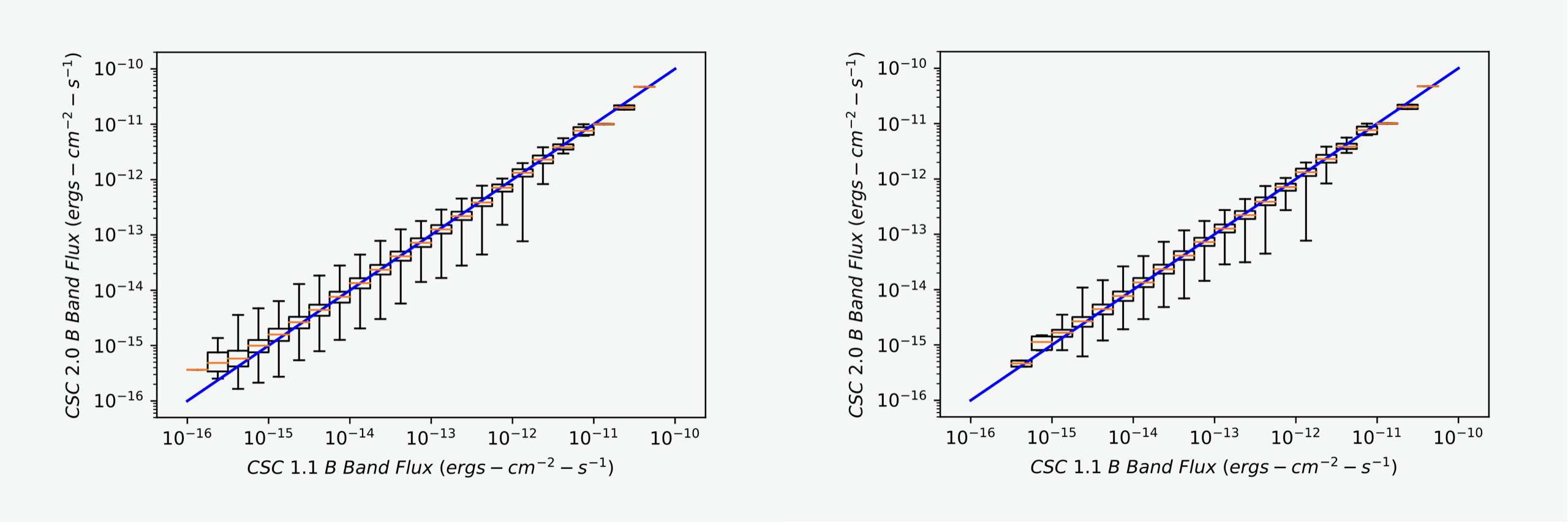### Figure 20: CSC 2.0 vs. CSC 1.1 Master Source Fluxes

Comparison of CSC 1.1 and CSC 2.0 master source fluxes for the full sample (left) and the high-significance sample (right). In each flux bin, boxes represent the inter-quartile range (25%–75% percentiles), while the bars above and below encompass 99% of the points and the red horizontal lines indicate the medians. The blue lines indicate the locus of points where CSC 1.1 and CSC 2.0 fluxes are equal. Each flux bin between 10-15 and 10-13 ergs cm-2 s-1 contains ∼2000–10000 points.

To examine the differences in more detail, we compute curves of the fraction of sources in the samples for which the percent difference between CSC 1.1 and CSC 2.0 fluxes is ≤10, 20, or 50%. Results are shown in Figure 21. In both samples, the percent difference is ≤∼50% for most sources brighter than ∼2⨯10-15 ergs cm-2 s-1, while approximately half of the sources have percent differences less than ∼10% for fluxes brighter than ∼10-14 ergs cm-2 s-1.

### Figure 21: CSC 2.0 vs. CSC 1.1 Master Source Fluxes Percentage Difference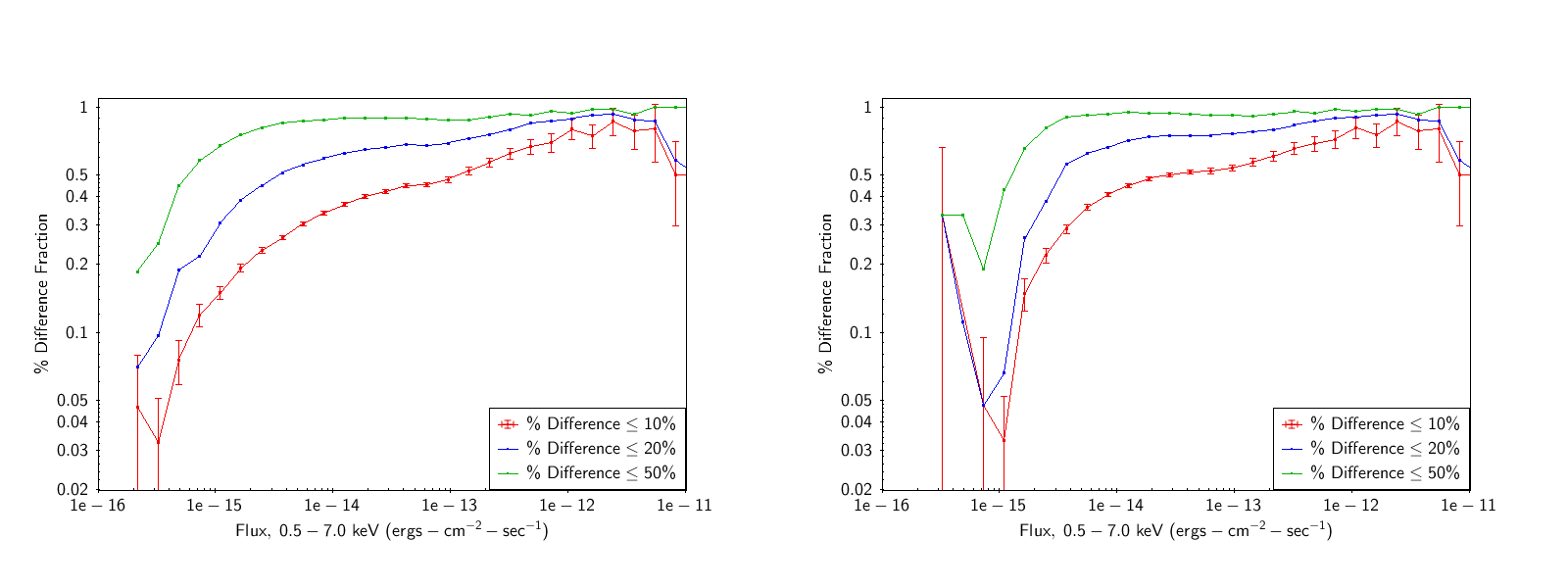### Figure 21: CSC 2.0 vs. CSC 1.1 Master Source Fluxes Percentage Difference

Percent differences between CSC 1.1 and CSC 2.0 master source fluxes for the full sample (left) and the high-significance sample (right). In each flux bin, the fraction of sources with percent differences ≤10, 20, and 50% are plotted. Typical $$\sqrt{n}$$ error bars are plotted for the 10% curves.

We are continuing to investigate these effects. We note that although the data are the same for both CSC 1.1 and CSC 2.0 sources in the two samples, the calibration data, most notably the effective areas, may differ due to the evolution of the ACIS contamination model. There are also subtle differences between the aperture photometry algorithms used in CSC 1.1 and CSC 2.0.

## Source Size

The observed spatial distribution of events from a source is the convolution of the source's intrinsic spatial distribution and the PSF. CSC 2.0 uses a Mexican-Hat optimization algorithm to estimate the intrinsic source size from the observed size and the PSF size (see Source Extent and Errors). Master sources are classified as extended if the observed size is inconsistent with the PSF size at the 90% confidence level in any of the contributing observations or stacks, in any band. In a preliminary sample of ∼91,000 master sources, ∼8% are flagged as extended, with the percentage being slightly larger for TRUE sources (∼8.3%) than for MARGINAL sources (∼7.0%). This may be a selection effect, since MARGINAL sources tend to have fewer counts than TRUE sources, and are thus less likely to have statistically significant extent measurements. For both TRUE and MARGINAL sources, the flux distributions for extended sources are skewed toward higher values, as indicated in Figure 22.

### Figure 22: Normalize Flux Distributions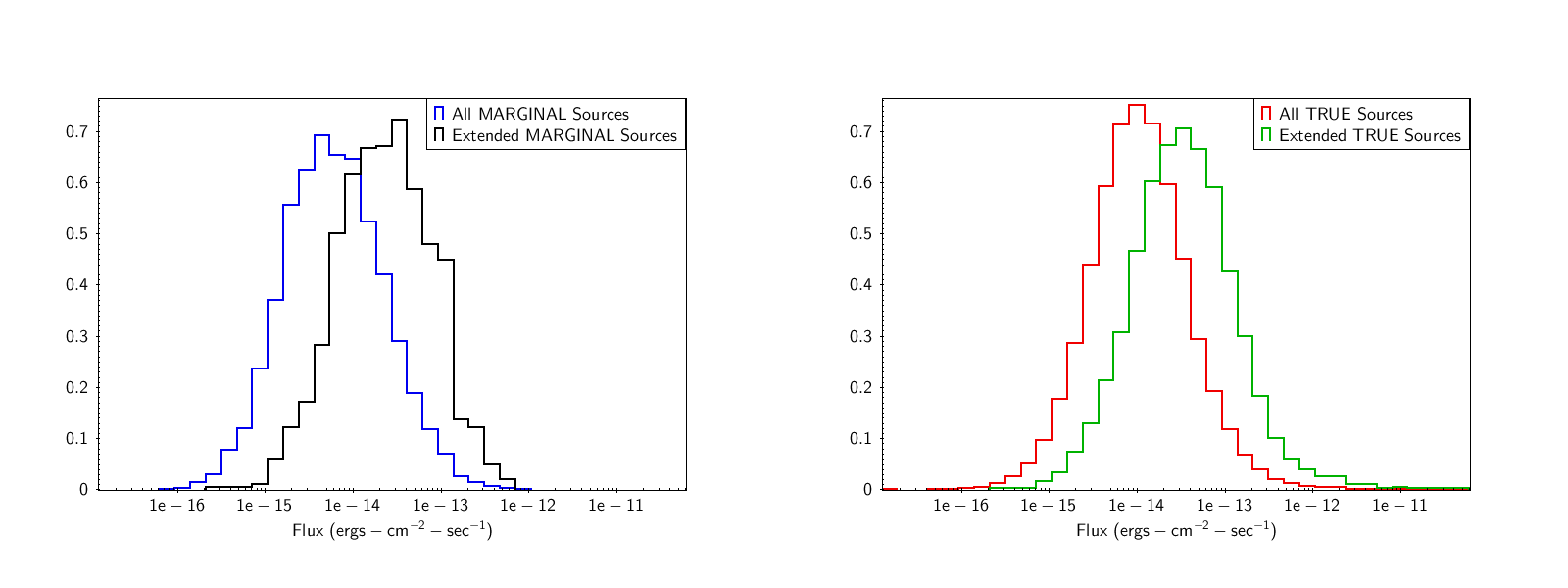### Figure 22: Normalize Flux Distributions

Normalized distributions of fluxes for extended and all sources classified as MARGINAL (left) and TRUE (right). The energy range for the fluxes is 0.5-7.0 keV (b-band) for ACIS observations, and 0.1-10 keV (w-band) for HRC observations.

CSC 2.0 models extended sources as elliptical Gaussian distributions and we define source size

$\sigma_{ext}=\sqrt{\sigma_{major}\sigma_{minor}} \ ,$

where $$\sigma_{major}$$ and $$\sigma_{minor}$$ are the values of $$\sigma$$ along the major and minor axes of the Gaussian distribution. The overall distribution of $$\sigma_{ext}$$ for extended sources is shown in Figure 23, and ranges from ∼0.1″–∼100″. As in CSC 1.1, there is a trend toward larger measured source sizes at larger values of $$\theta$$, for both extended and unextended sources, indicating that our current source extent algorithm can only weakly discriminate between actual extent and the large asymmetric PSFs of point sources at large values of $$\theta$$.

### Figure 23: Distribution of Source Size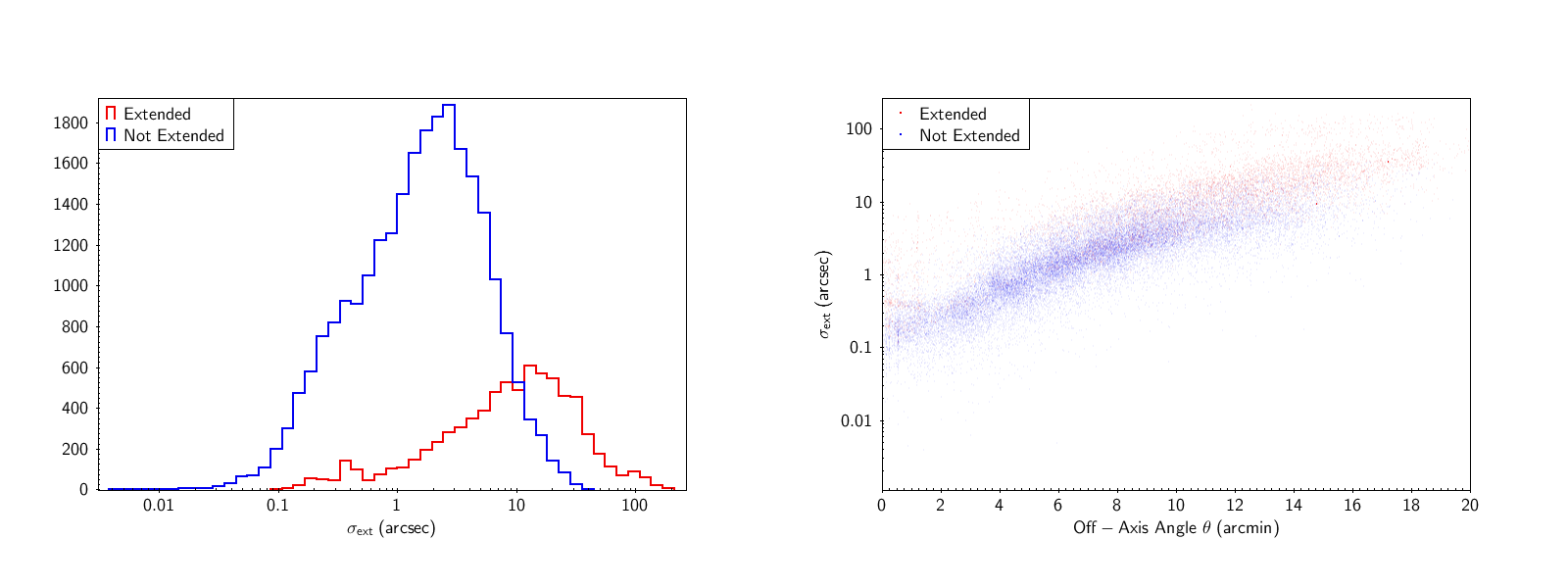### Figure 23: Distribution of Source Size

Left: Distribution of source size for sources classified as extended (red) and unextended (blue). Right: Dependence of source size on off-axis angle $$\theta$$.

Finally, to investigate systematic errors in classifying sources as extended, we examine the extent information in our astrometric sample of CSC 2.0-SDSSDR13 stars, under the assumption that these sources should all be unextended. The fraction of sources (erroneously) classified as extended is shown in Figure 24, as a function of off-axis angle $$\theta$$. This fraction is more than ∼10% for $$\theta>\sim10^{\prime}$$ and falls to below ∼1% for $$\theta<\sim5^{\prime}$$. However, there appears to be an excess for sources that are nearly on-axis. We attribute this to an excess sharpness in current on-axis PSF models (see, e.g., MARX Accuracy and Testing: Point Spread Function).

### Figure 24: Extended Source Fractions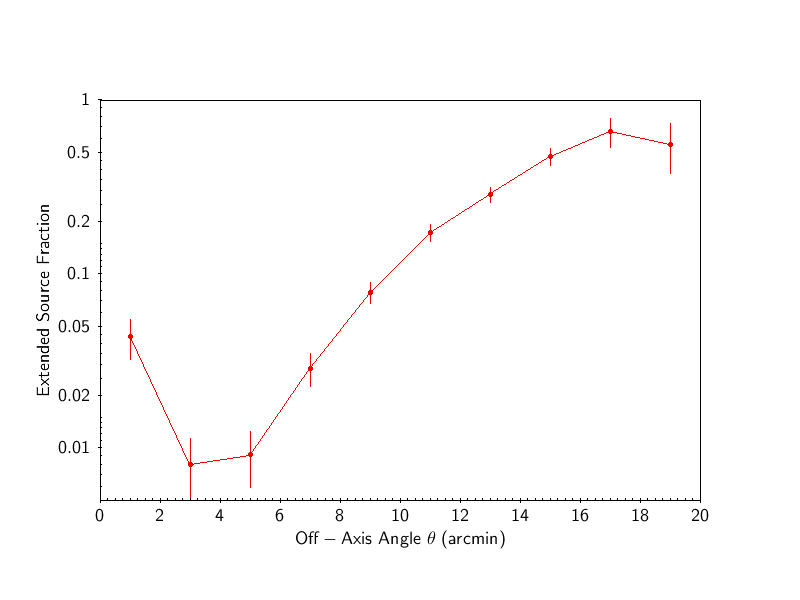### Figure 24: Extended Source Fractions

Fraction of sources classified as extended in a sample of CSC 2.0 sources matched with SDSS DR13 stars.

## Variability

### Inter-Observation Variability

As described in the Source Variability column descriptions, if a source is observed in multiple observations, we estimate the probability that the source photon flux varied among the contributing observations, based on a likelihood ratio test. We also compute a variability index, similar to that used to describe intra-observation variability.

To investigate these properties we examined ∼68,000 master sources observed in ∼276,000 observations (excluding upper limits) in the b-band, and computed

$\chi^2_{\nu} = \frac{\sum_{i=1}^{n_{obs}} \frac{\left(\mathit{photflux\_aper\_b_{i}} - \mathit{photflux\_aper\_avg\_b}\right)^{2}} {\sigma_{\mathit{photflux\_aper\_b_{i}}}^{2}}} {n_{obs}-1}$

where

$\sigma_{\mathit{photflux\_aper\_b_{i}}} = \frac{\mathit{photflux\_aper\_hilim\_b_{i}}-\mathit{photflux\_aper\_lolim\_b_{i}}} {2} \ .$

A plot of $$\chi^{2}_{\nu}$$ vs. Inter-Observation Probability is shown in Figure 25.

### Figure 25: Inter-Observational Variability Probability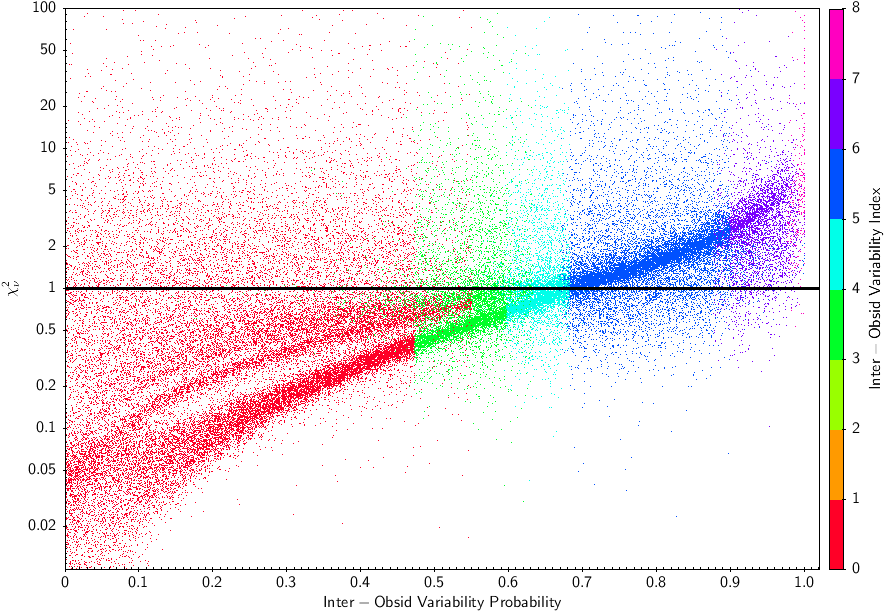### Figure 25: Inter-Observational Variability Probability

Reduced $$\chi^{2}$$, assuming constant photon flux, vs. Inter-Observation Probability, for a sample of ∼68,000 master sources observed in multiple observations in the b-band. Inter-Observation Variability Index values are also shown.

### Intra-Observation Variability

The Chandra Source Catalog utilizes three variability tests: Kolmogorov-Smirnov, Kuiper, and Gregory-Loredo. Results from these tests are stored as a probability, $$p$$, that the lightcurve in the given band for the indicated variability test is not consistent with being constant (i.e., pure counting noise, modulo source visibility/good time intervals).

For purposes of characterization, a more useful probability is $$P=1-p$$, which can be taken as the probability that a lightcurve would have indicated the detected level of variability. It is further convenient to take the negative $$\log_{10}$$ of this quantity, or equivalently, $$\log_{10}\left( P^{-1} \right)$$. For much of the characterization that follows, results are presented in terms of this quantity.

Note that for pure counting noise (i.e., constant) simulated lightcurves, we expect for a "good" test that the fraction $$f_{P}$$ of lightcurves that are detected as variable at a high significance (e.g. 99%) will be small, for rates and fractional root mean square (RMS) noise levels that are within the expected observed values.

To assess the sensitivity of the variability tests, we have (outside of the source catalog pipeline) created a series of simulated lightcurves with differing durations (from 1 ksec to 160 ksec, utilizing 3.214 sec time bins), mean count rates (ranging from 0.00056 to 0.032 counts per second), and different variability properties. Additionally, we have incorporated a simple model of pileup in the simulations, such that if two or more events occur in the same time bin there is a probability that the event will be discarded, or read as a single event.

First, we investigated the sensitivity to "red noise", i.e., variability with a power spectrum that is proportional to Fourier frequency $$f^{-1}$$. (The frequency range is assumed to cover from the inverse of the lightcurve length to the Nyquist frequency.) The lightcurves were presumed to be statistically stationary, and a variety of fractional (RMS) variabilities were considered, ranging from 1% to 30%.

In Figures 26–28, we show "detection contours" vs. mean rate and fractional RMS variability for the Kolmogorov-Smirnov, Kuiper, and Gregory-Loredo tests, for three different lightcurve lengths of 20 (left panel), 50 (middle panel), and 160 (right panel) kiloseconds. The contours show the $$f_{P}$$, the fraction of simulations whose lightcurves yielded $$P < 0.01$$, or equivalently $$\log_{10}\left( P^{-1} \right) > 2$$, for the given test. Note that such small value of $$P$$ would imply variability at the 99% confidence level. These curves give an indication of the sensitivity of the test to aperiodic, red noise variability.

Take, for example, the Kolmogorov-Smirnov test. Note that for the 20 ksec observations, at any simulated photon rate, only a small fraction (<1%) of these lightcurves are detected as variable below a 10% RMS noise level. We need to get almost to the 30% RMS noise level before a significant fraction of the non-variable lightcurves are detected as variable by the K-S test. This fraction increases with exposure time. This is expected, as for the same signal, the chance of fluctuations high enough to trigger the variability test increases with exposure.

### Figure 26: Fraction of Source Variability (Kolmogorov-Smirnov test)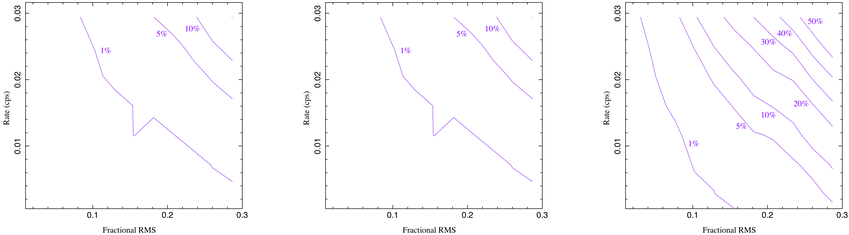### Figure 26: Fraction of Source Variability (Kolmogorov-Smirnov test)

Fraction of simulated sources detected as variable at 99% significance, using the Kolmogorov-Smirnov test.

### Figure 27: Fraction of Source Variability (Kuiper test)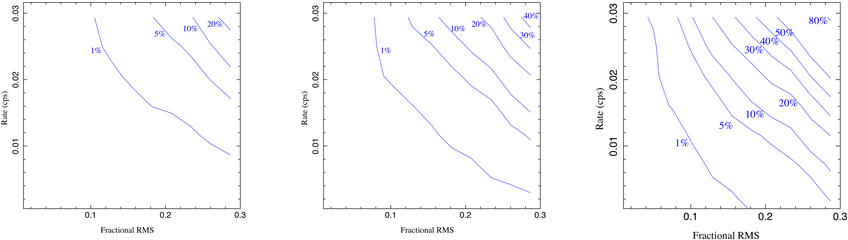### Figure 27: Fraction of Source Variability (Kuiper test)

Fraction of simulated sources detected as variable at 99% significance, using the Kuiper test.

### Figure 28: Fraction of Source Variability (Gregory-Loredo test)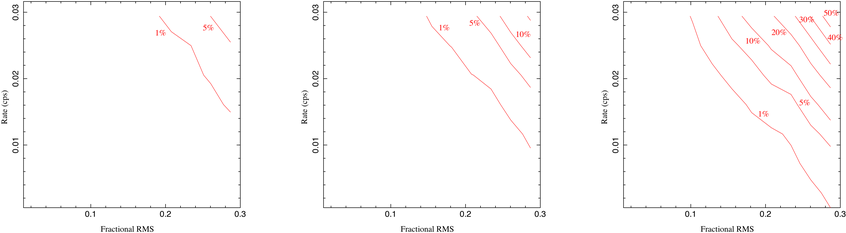### Figure 28: Fraction of Source Variability (Gregory-Loredo test)

Fraction of simulated sources detected as variable at 99% significance, using the Gregory-Loredo test.

Finally, we show histograms of cumulative fraction of lightcurves detected with a significant variability probability (above some value of $$\log_{10}\left( P^{-1} \right)$$). Again, for pure Poisson counting noise, we expect that this cumulative fraction will follow $$P$$. In Figure 29 we show the expected histogram for no variability as an orange line. Cumulative fraction histograms are shown for a variety of lightcurve lengths, mean count rates, and fractional root mean square variability. Note that, for a given confidence level (e.g. $$\log_{10}\left( P^{-1} \right) = 2$$), the fraction of sources detected as variable increases with the level of RMS noise variability, going significantly above the "non-variability" expected fraction only for high RMS variability. Again, the fraction of false alarm detections increases with increasing exposure time. Of all three tests, the Gregory-Loredo test appears to be the most sensitive to changes in RMS noise.

### Figure 29: Cumulative fraction of simulated lightcurves detected with a significant probability of variability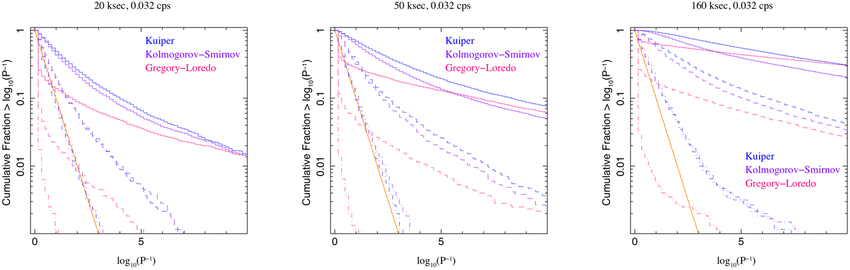### Figure 29: Cumulative fraction of simulated lightcurves detected with a significant probability of variability

Cumulative fraction of simulated lightcurves detected with a significant probability of variability. The orange histogram is that expected for no variability, assuming Poisson noise. Three sets of RMS variability are shown: 30% (solid histogram), 15% (longdash), and 5% (dot-dash).